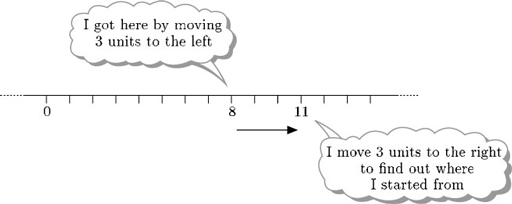Numbers, units and arithmetic

This free course is available to start right now. Review the full course description and key learning outcomes and create an account and enrol if you want a free statement of participation.

Free course

# 3.17 Subtraction of negative numbers

Next consider subtraction of a negative number. In terms of Thomas’s piggy bank, subtracting a negative number is the same as taking away one of his IOUs. If his mother says ‘you have been a good boy today so I’ll take away that IOU for £3’ this is equivalent to him being given £3.

So, − (3) = 3. Does this correspond with the number line interpretation of subtracting a negative number?

Consider the evaluation of 8 − 3. Continue to think of subtraction as undoing addition: adding 3 to 11 gives 8, and so subtracting3 from the answer, 8, gets back to 11. So, in terms of the number line, subtracting 3 from 8 means starting at 8 and moving 3 units to the right.But moving 3 units to the right is the same as adding 3. So

8 − 3 = 8 + 3 = 11.

If on one occasion Thomas's piggy bank is worth £8 in total (cash and IOUs) and his mother takes away a £3 IOU, the value of the piggy bank is 8 − 3 = 8 + 3 = 11.

Subtracting a negative number is the same as adding the corresponding positive number.

MU120_4M1